Courses

# Test: Helical Springs - 2

## 8 Questions MCQ Test Topicwise Question Bank for Mechanical Engineering | Test: Helical Springs - 2

Description
This mock test of Test: Helical Springs - 2 for Mechanical Engineering helps you for every Mechanical Engineering entrance exam. This contains 8 Multiple Choice Questions for Mechanical Engineering Test: Helical Springs - 2 (mcq) to study with solutions a complete question bank. The solved questions answers in this Test: Helical Springs - 2 quiz give you a good mix of easy questions and tough questions. Mechanical Engineering students definitely take this Test: Helical Springs - 2 exercise for a better result in the exam. You can find other Test: Helical Springs - 2 extra questions, long questions & short questions for Mechanical Engineering on EduRev as well by searching above.
QUESTION: 1

### Which one of the following is the correct expression for maximum shear stress induced in the wire of a closed-coiled helical spring of wire diameter d and mean coil radius R which carries an axial load W?

Solution:

From the equation of torsion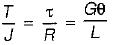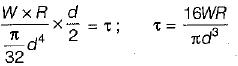QUESTION: 2

### Given that, d = diameter of spring, R = mean radius of coils, n = number of coiis and G = modulus of rigidity, the stiffness of the close-coiied helical spring subject to an axial load W is equal to

Solution:

The deflection of a close coil helical spring subjected to axial  force ‘W’ is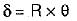Where δ = Deflection of spring θ = angle of twist
= TL/GJ
∴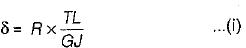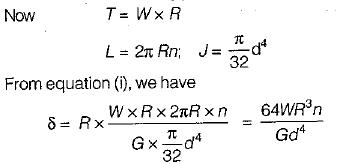Spring constant or stiffness factor is defined as the axial force required to produce unit deflection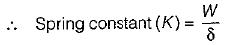∴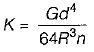QUESTION: 3

### A closely coiled helical spring of radius R, contains n turns and is subjected to an axial load W. if the radius of the coil wire is r and modulus of rigidity of the coil material is G, the deflection of the coil is

Solution:

∴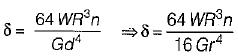⇒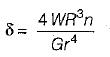QUESTION: 4

Consider the following types of stresses:
1. Torsional shear
2. Transverse direct shear
3. Bending stress

The stresses that are produced in the wire of a closed coiled helical spring subjected to an axial load, would include

Solution:

When axial load is applied to the spring stress are 3etup due to:
(i) Torsion
(ii) Direct shear and
(iii) Bending
Where ever the stresses due to direct shear and bending are very small and may be neglected.

QUESTION: 5

A closed-coil helical spring is subjected to a torque about its axis. The spring wire would experience a

Solution:

When a closed coil helical spring, fixed at one end is subjected to a twisting couple about the central axis of the spring, then bending moment will be produced at every cross-section of the spring. The twisting couple will try to wind up or unwind the spring.

QUESTION: 6

A weighing machine consists of a 2 kg pan resting on a spring, in this condition, with the pan resting on the spring, the length of the spring is 200 mm. When a mass of 20 kg is placed on the pan, the length of the spring becomes 100 mm? For the spring, the un-deformed length L0 and the spring constant k (stiffness) are

Solution: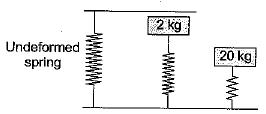Stiffness of the spring will be same in each case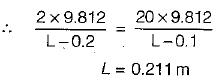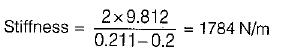QUESTION: 7

A close-coiled helical spring shown below in figure-I is to be cut into two equal pieces and combined as a parallel spring as shown in figure-II. The ratio of the maximum angular twist of the situation shown in figure-II to that of figure-I, due to the same load W will be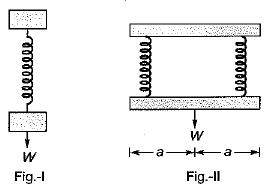Solution:

Angular twist in spring is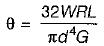Where,
d is diameter of wire of spring
G is modulus of rigidity
W is axial pull on spring
R is mean radius of coil
L is length of spring
∴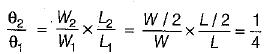QUESTION: 8

A close-coiled helical spring has wire diameter 10 mm and spring index 5. If the spring contains 10 turns, then the length of the spring wire would be

Solution:

Wire diameter, d = 10 mm
Spring index = 5 = D/d
D = 5d; D = 50 mm
Number of turns n = 10
Length of wire = πD x n = π x 50 x 10 = 3.14 x 500 = 1570 mm